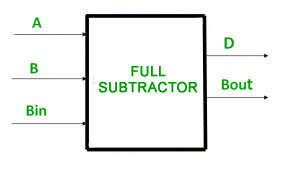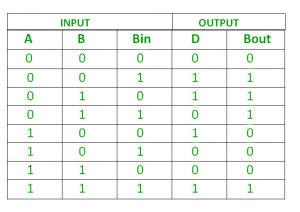# Python Program to implement Full Subtractor

• Last Updated : 21 Nov, 2021

Prerequisite –Full Subtractor in Digital Logic

In this, we will discuss the overview of the full subtractor and will implement the full subtractor logic in the python language. Also, we will cover with the help of examples. Let’s discuss it one by one.

Given three inputs of Full Subtractor A, B, Bin. The task is to implement the Full Subtractor circuit and Print output i.e Difference (d) and B-Out of three inputs.

Full Subtractor :

The full Subtractor is a combinational circuit that is used to perform subtraction of three input bits: the minuend, subtrahend, and borrow in. The full Subtractor generates two output bits: the difference and borrow out.Logical Expression :

```Difference = (A XOR B) XOR Bin
Borrow Out = Ā Bin + Ā B + B Bin```

Truth Table :Examples :

```Input       : 0 1 1
Output      : Difference=0, B-Out=1
Explanation : According to logical expression Difference= (A XOR B) XOR Bin  i.e (0 XOR 1) XOR 1  =0 ,
B-Out=Ā Bin + Ā B + B Bin  i.e  1 AND 1 + 1 AND 1 + 1 AND 1 = 1

Input       : 1 0 0
Output      : Difference=1, B-Out=0```

Approach :

• We take three inputs A, B, and Bin.
• Applying (A XOR B) XOR Bin gives the Value of Difference.
• Applying Ā Bin + Ā B + B Bin gives the value of B-Out.

Below is the implementation :

## Python3

 `# python program to implement full Subtractor` `# Function to print Difference and B-Out`   `def` `getResult(A, B, ``Bin``):`   `    ``# Calculating value of Difference` `    ``Difference ``=` `(A ^ B) ^ ``Bin` `    `  `    ``# calculating NOT  value of a` `    ``A1 ``=` `not``(A)` `    `  `    ``# Calculating value of B-Out` `    ``B_Out ``=` `A1 & ``Bin` `| A1 & B | B & ``Bin`   `    ``# printing the values` `    ``print``(``"Difference = "``, Difference)` `    ``print``(``"B-Out = "``, B_Out)`     `# Driver code` `A ``=` `0` `B ``=` `1` `Bin` `=` `1` `# passing three inputs of fullsubtractor as arguments to get result function` `getResult(A, B, ``Bin``)`

Output :

```Difference =  0
B-Out      =  1```

Attention geek! Strengthen your foundations with the Python Programming Foundation Course and learn the basics. To begin with, your interview preparations Enhance your Data Structures concepts with the Python DS Course.

My Personal Notes arrow_drop_up
Recommended Articles
Page :